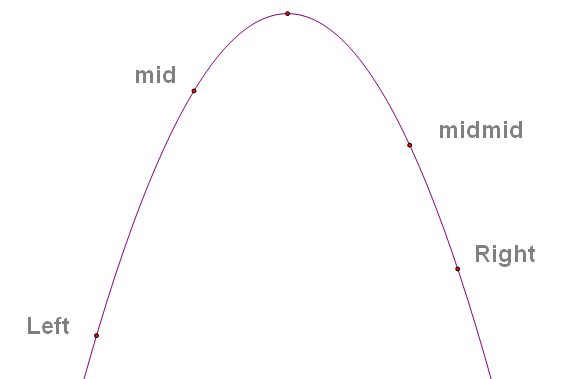lethic@163.com.LeftRightmid = (Left + Right) / 2midmid = (mid + Right) / 2; midRight = midmid(midmid)Left = mid;

double Calc(Type a)
{
/* */
}
void Solve(void)
{
double Left, Right;
double mid, midmid;
double mid_value, midmid_value;
Left = MIN; Right = MAX;
while (Left + EPS < Right) { mid = (Left + Right) / 2; midmid = (mid + Right) / 2; mid_area = Calc(mid); midmid_area = Calc(midmid); // . if (mid_area >= midmid_area) Right = midmid;
else Left = mid;
}
}
OJ
buaa 1033 Easy Problem
http://acm.buaa.edu.cn/oj/problem_show.php?c=0&p=1033

CalcP
ZOJ 3203 Light Bulb
http://acm.zju.edu.cn/onlinejudge/showProblem.do?problemCode=3203